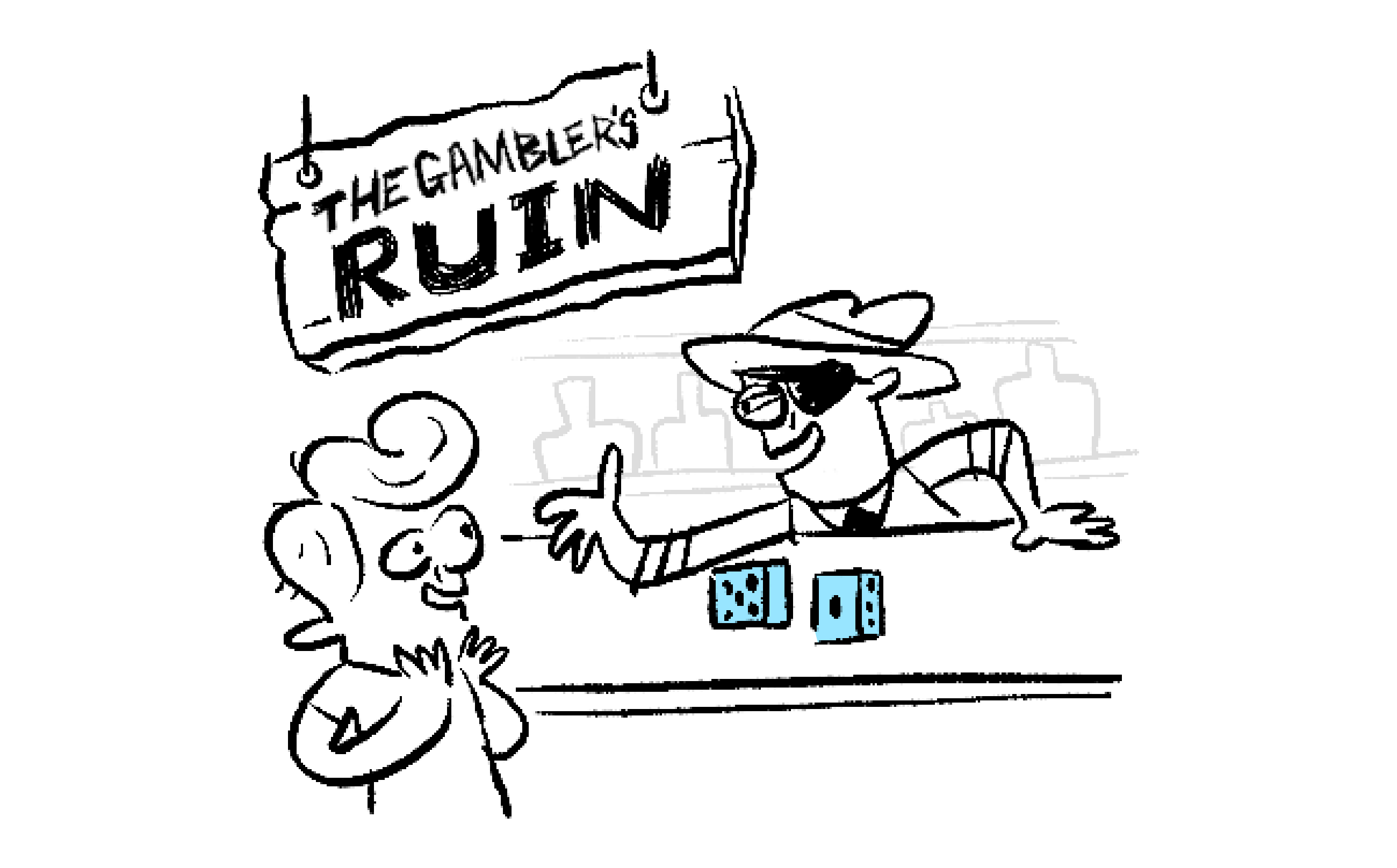### Statistics I

The central limit theorem, or CLT for short, is absolutely vital to statistics, so it'll crop up many times throughout our course.

In a nutshell, the CLT says that the sum of a large number of random draws is roughly distributed like a bell curve.

This is a casual version of the true CLT, but it's catchy and suits our needs well enough.

In this quiz, we'll unpack this statement and uncover the intuitive ideas at the heart of the CLT.

Without further ado, let's begin building our CLT intuition with a trip to the casino...

# Roll the Dice: The Central Limit Theorem

Many fortunes have been lost at the tables of The Gambler's Ruin casino, but Marvin isn't thinking about that: he's too intent on having some fun and, hopefully, winning some cash.

Fortunately for him, his wiser and savvier friend Zhang Wei tags along to make sure Marvin doesn't gamble away all of his savings.

The pair descend onto the main floor of The Gambler's Ruin and immerse themselves in a cacophony of sounds and a galaxy of flashing lights.

Zhang Wei guides his friend quickly past the rows of slot machines and roulette wheels to a game called "Roll of the Dice," where even gullible Marvin may just have a shot at winning...# Roll the Dice: The Central Limit Theorem

"The game is simple," explains the croupier. "You pick a number, and then wager $\1$ that the dice will roll that value."

The croupier looks Marvin up and down and decides he looks a bit of a rube, so she decides to go easy on him at first.

"We'll start with a single die, so you can bet on a roll of $1, 2, 3, 4, 5,$ or $6.$ What's your bet?"

Marvin pauses, scratches his head, and gives serious consideration to the options before him.

If the die is fair, what's Marvin's best strategy for winning?

# Roll the Dice: The Central Limit Theorem

Zhang Wei stands off to the side and watches as Marvin makes his first bet... and loses.

"Tough break," says the croupier with a hint of mock sympathy. "Better luck next time! Want to try a more exciting game?"

Marvin nods and listens as the croupier explains the rules. "This time, I throw two dice and you bet on the total value of the two rolls. So, if you bet on $11$ and one die comes up $5$ and the other comes up $6,$ you win; otherwise, you lose your wager. Got it?"

Marvin nods again and thinks over his options. He turns to Zhang Wei and says "$6$ and $12$ are two of my lucky numbers. What do you think? Should I bet on $6$ or $12?$"

Assuming the dice are fair and the rolls are independent, what's Zhang Wei's best advice to Marvin to maximize his chances of winning?

Hint: A throw of the dice can be represented as a pair of integers, so the sample space is \begin{aligned} \big\{ & (1,1), (1,2), (1,3),(1,4),(1,5),(1,6) \\ & (2,1), (2,2), (2,3),(2,4),(2,5),(2,6) \\ & (3,1), (3,2), (3,3),(3,4),(3,5),(3,6) \\ & (4,1), (4,2), (4,3),(4,4),(4,5),(4,6) \\ & (5,1), (5,2), (5,3),(5,4),(5,5),(5,6) \\ & (6,1), (6,2), (6,3),(6,4),(6,5),(6,6) \big\}. \end{aligned}# Roll the Dice: The Central Limit Theorem

Marvin takes Zhang Wei's advice, bets on $6,$ and ends up winning!

The croupier smiles, gives a few words of encouragement, and then invites Marvin to up the challenge by betting on the total roll of three dice.

He's just about through pondering his choices when he feels a tap on his shoulder. Marvin turns and sees Zhang Wei holding out his phone to him. On the screen is the following interactive plot:

Zhang Wei explains that a bar's height in this histogram represents the number of ways $n$ dice can roll a sum total of $S_{n},$ the integer below it.

For example, there's only one way to roll a $3,$ namely $(1,1,1),$ but there are $27$ different ways of rolling an $11;$ that's why its bar is so much higher than $3$'s.

Given Zhang Wei's histogram and the assumption that the dice are all fair and the rolls are independent, how should Marvin bet?

# Roll the Dice: The Central Limit Theorem

The croupier adds one more die to the roll after every bet to make the game more fun, but she's really just making it harder for Marvin to win by adding more possible outcomes.

Zhang Wei suspected she'd do this.

Fortunately, he came prepared: the plot he shares with Marvin has a slider labeled $n$ for the number of dice used in a roll (see below).

Zhang Wei adjusts the scale by dividing the heights of the histogram bars by $6^n$ so the plot displays the probability distribution for $S_{n},$ the sum total of a roll of $n$ fair independent dice: \small \begin{aligned} P(n \text{ dice roll a total of } S_{n} ) = \frac{\text{(number of ways } n \text{ dice can sum to } S_{n}) }{6^{n}}. \end{aligned} Change the value of $n$ and study the shape of the distributions as you do. What do you notice?